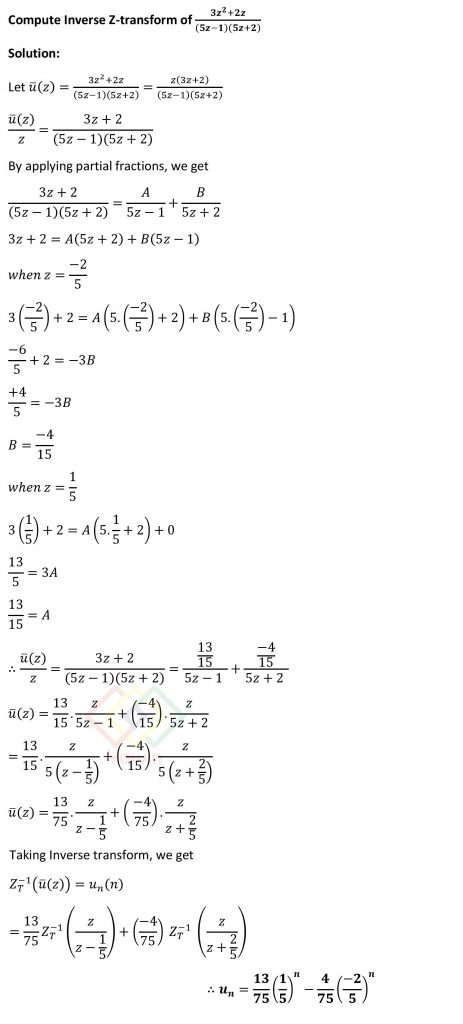Compute Inverse Z-transform of (3z^2+2z)/ ((5z-1) (5z+2))

## Question## Question

Compute Inverse Z-transform of (3z^2+2z)/ ((5z-1) (5z+2))